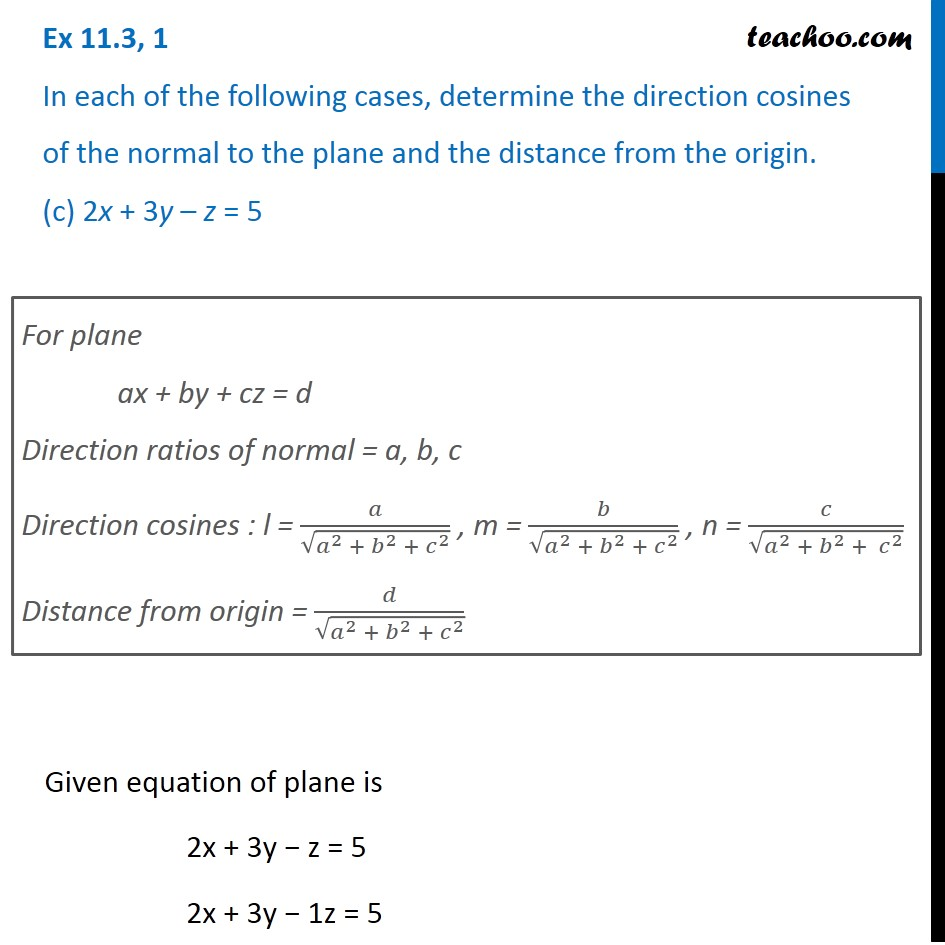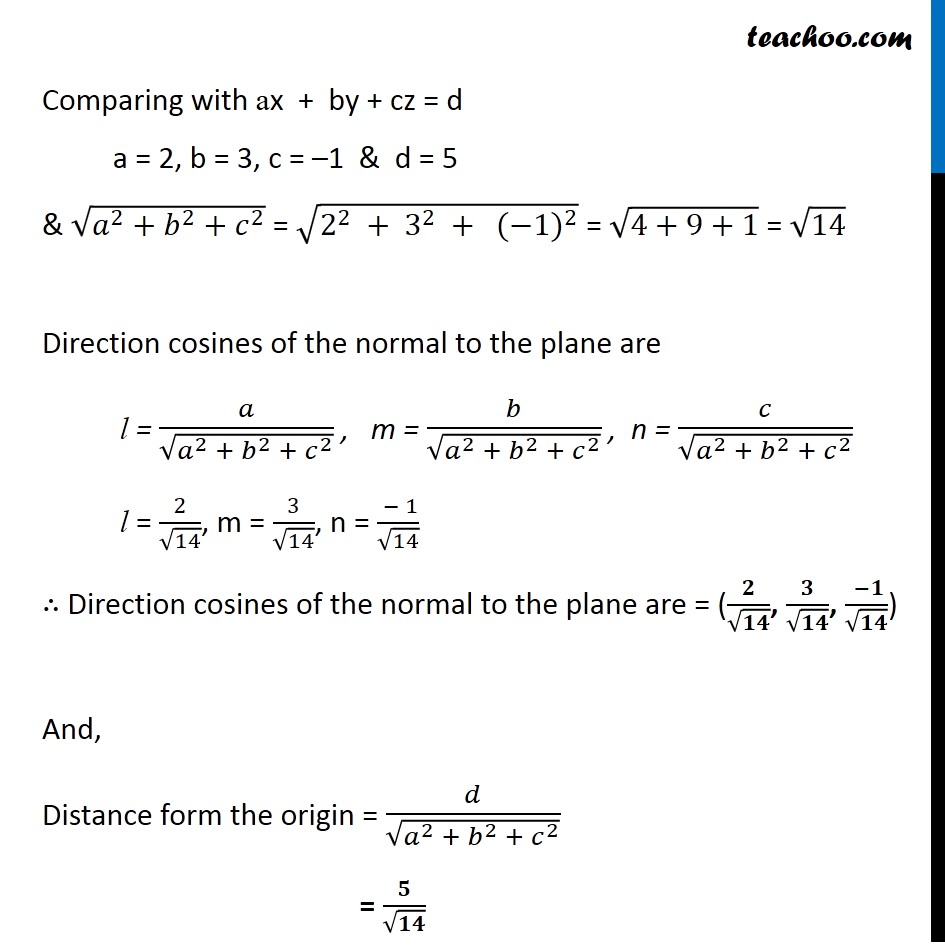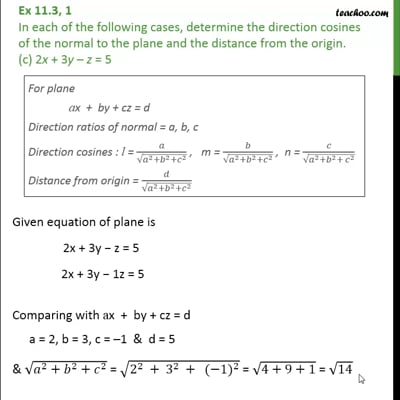Ex 11.3

Chapter 11 Class 12 Three Dimensional Geometry
Serial order wiseThis video is only available for Teachoo black users

Maths Crash Course - Live lectures + all videos + Real time Doubt solving!

### Transcript

Ex 11.3, 1 In each of the following cases, determine the direction cosines of the normal to the plane and the distance from the origin. (c) 2x + 3y – z = 5 For plane ax + by + cz = d Direction ratios of normal = a, b, c Direction cosines : l = 𝑎/√(𝑎^2 + 𝑏^2 +〖 𝑐〗^2 ) , m = 𝑏/√(𝑎^2 + 𝑏^2 + 𝑐^2 ) , n = 𝑐/√(𝑎^2 + 𝑏^2 + 𝑐^2 ) Distance from origin = 𝑑/√(𝑎^2 + 𝑏^2 + 𝑐^2 ) Given equation of plane is 2x + 3y − z = 5 2x + 3y − 1z = 5 Comparing with ax + by + cz = d a = 2, b = 3, c = –1 & d = 5 & √(𝑎^2+𝑏^2+𝑐^2 ) = √(2^2 + 3^2 + 〖(−1)〗^2 ) = √(4+9+1) = √14 Direction cosines of the normal to the plane are l = 𝑎/√(𝑎^2 + 𝑏^2 + 𝑐^2 ) , m = 𝑏/√(𝑎^2 + 𝑏^2 + 𝑐^2 ) , n = 𝑐/√(𝑎^2 + 𝑏^2 + 𝑐^2 ) l = 2/√14, m = 3/√14, n = ( − 1)/√14 ∴ Direction cosines of the normal to the plane are = (𝟐/√𝟏𝟒, 𝟑/√𝟏𝟒, ( −𝟏)/√𝟏𝟒) And, Distance form the origin = 𝑑/√(𝑎^2 + 𝑏^2 +〖 𝑐〗^2 ) = 𝟓/√𝟏𝟒